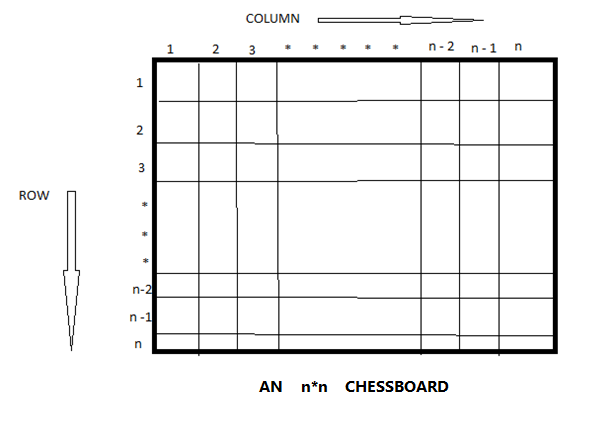# N Queen's problem and solution using backtracking algorithm

In this article, we are going to learn about the N Queen's problem and how it can be solved by using backtracking?
Submitted by Shivangi Jain, on June 29, 2018

## N - Queen's problem

The n – queen problem is the generalized problem of 8-queens or 4 – queen’s problem. Here, the n – queens are placed on a n * n chess board, which means that the chessboard has n rows and n columns and the n queens are placed on thus n * n chessboard such that no two queens are placed in the same row or in the same column or in same diagonal. So that, no two queens attack each other.

Here, we suppose that the queen i is to be placed in row i. We can say that 1 queen is placed in the first row only but can have columns from 1, 2... n so that they satisfy all the explicit and implicit constraints.

All solutions to the n – queen’s problem can be therefore represented as n – tuples (x1, x2... xn) where xi is the column on which queen i is to be placed.

The explicit constraints using this formulation are Si = {1, 2, 3... n-1, n}, where 1 <= I <= n. Therefore, the solution space consists of nˆn n- tuples. Now, while considering the implicit constraints, that no two xi’s can be same i.e., two queens cannot be in the same row, same column, or in same diagonal. So, each xi should be different. Now by the above constraint, the solution is the permutations of the n – tuples (1, 2, 3, 4... n-1, n).

### Algorithm

For all the solutions of the n - queen’s problem...

```1.	Algorithm N Queen (k, n)
2.	// Using backtracking, this procedure prints all possible placements of
3.	// n- queens on the n*n chess board so that they are non-attacking.
4.	{
5.	For I = 1 to n do
6.	{
7.	If Place (k, i) then
8.	{
9.	X[k] = I;
10.	If (k = n) then write (x[1: n ]) ;
11.	 Else N Queens (k+1, n);
12.	}
13.	}
14.	}
```What's New

Top Interview Coding Problems/Challenges!

Languages: » C » C++ » C++ STL » Java » Data Structure » C#.Net » Android » Kotlin » SQL
Web Technologies: » PHP » Python » JavaScript » CSS » Ajax » Node.js » Web programming/HTML
Solved programs: » C » C++ » DS » Java » C#
Aptitude que. & ans.: » C » C++ » Java » DBMS
Interview que. & ans.: » C » Embedded C » Java » SEO » HR
CS Subjects: » CS Basics » O.S. » Networks » DBMS » Embedded Systems » Cloud Computing
» Machine learning » CS Organizations » Linux » DOS
More: » Articles » Puzzles » News/Updates